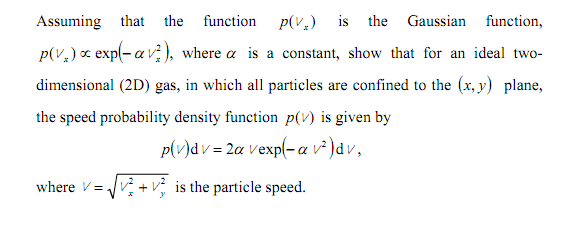# The Maxwell Speed Distribution in 2D

fatherdaly

## Homework StatementIt seemed much easier to screencap than to write out.

## Homework Equations

It helps to know that the number of states with speed between u and u+du is 2pi*u du

## The Attempt at a Solution

I've tried quite a few things but every time I get to trying to normalise I either get stuck integrating by parts over and over, or using http://en.wikipedia.org/wiki/Gaussian_integral" [Broken]<that, which doesn't arrive at the answer wanted.

If someone could give me a push in the right direction it would be much appreciated.

Last edited by a moderator:

Ok so I have the integral of v*exp(-$$\alpha$$v2) dv between 0 and infinity to normalise. I don't know how a substitution would help because you would still have two functions multiplied by one another.